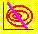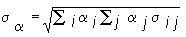6.5 THREE-FIRM CASE: ADDING A RISK-FREE ASSET

Suppose you are considering a portfolio of three securities, and you are permitted to borrow and lend at 12%. The portfolio in Table 6.1 provides an expected return of 14%, as derived in Chapter 4, in the topic titled Three-Firm Case: Application of Mean Variance Theory:

 Table 6.1: Minimum-Variance Portfolio Weights for an Expected Return = 14%.

 Portfolio Weights Firm 1 0.41 Firm 2 0.18 Firm 3 0.41

Call this Portfolio I. Now consider the set of portfolios that can be attained by combining Portfolio I with the risk-free asset. Returns from such portfolios are described by the straight line arf + (1- a)E(r) where proportion a of the portfolio is invested in the risk-free security and (1- a) is invested in Portfolio I. The proportion of total wealth that is allocated to risky assets in Portfolio I equals 41% in Firm 1, 18% in Firm 2, and 41% in Firm 3. Suppose your wealth is \$150,000, and you allocate \$100,000 to the three risky assets and \$50,000 to the risk-free asset. When this is the case a is 1/3 and (1-a) is 2/3. As a result, \$41,000 is invested in Firm 1, \$18,000 is invested in Firm 2, and \$41,000 is invested in Firm 3. This example provides an expected return equal to (1/3)12%+(2/3)14% = 13 1/3%.

Suppose your target return is 13%. This target can be attained in two ways. First, there exists a point on the minimum-variance (bullet-shaped) frontier that provides 13%. In CAPM Tutor it is easy to identify this portfolio by clicking on the appropriate part of the minimum-variance frontier. You may locate the appropriate portfolio this way (by trial and error) or by working through the analytics provided in Chapter 4, topic 4.9, titled Three-Firm Case: Application of Mean Variance Theory:

Firm 1 = 6.288E - 0.460

Firm 2 = 1.153E + 0.338

Firm 3 = 5.075E + 1.122

By letting E be 0.13, you can compute the resulting portfolio weights in Table 6.2.

 Table 6.2: Minimum-Variance Portfolio Weights for an Expected Return = 13%.
 Portfolio Weights Firm 1 0.349 Firm 2 0.188 Firm 3 0.462

This portfolio is Portfolio A in Figure 6.1 but we can consider an alternative portfolio that also has a 13% target return. This portfolio is engineered by combining the risk-free security and the risky Portfolio I by the appropriate choice of a. The weights of 1/3 and 2/3 provide a target return of 13 1/3%. To reach our new target of 13% we need more weight on the risk-free component and less weight on the risky component. That is, we want to choose a so that a rf + (1- a)E(r) equals .13. Since E(r) equals .14 and rf equals .12, clearly a must equal .5. In Figure 6.1, this is denoted as Portfolio B.

We now have two portfolios that provide an expected return equal to 0.13. The question that remains is: Does one portfolio dominate the other?

Notice in Figure 6.1. the different slopes of the line drawn between the risk-free security point (zero standard deviation, and expected return = 0.12) and portfolios A and B, respectively. The line through B has a greater slope than the line through A. This is because the expected return from B is 0.14 and the expected return from A is 0.13. Every point on the line through B (apart from rf itself) lies above every point on the line through A. The portfolio variance for the portfolio of half risk-free asset and half Portfolio B must be smaller than the portfolio variance for the portfolio of zero risk-free asset and only Portfolio A. Therefore, Portfolio B dominates Portfolio A.

Numerically, this can be verified by computing the portfolio standard deviation for both portfolios. This is computed as:The subscripts i,j are defined from 1 to 3 for the 3 securities.

For a review of this formula see Chapter 2, topic 2.9, Portfolio Statistics. Applied to the current example, the two portfolio standard deviations are:

 Portfolio Standard Deviation Portfolio A 0.1179 Portfolio B(.5 rf .5I) 0.0903

As a result, the second portfolio that exploits the existence of the risk-free security dominates Portfolio A.

So what is the best that you can do? This question is explored in topic 6.6, Capital Market Line.

previous topic

next topic